# 14-240/Tutorial-November4

## Boris

#### Question 26 on Page 57 in Homework 5

Let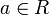$a \in R$ and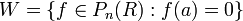$W = \{f \in P_n(R): f(a) = 0\}$ be a subspace of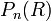$P_n(R)$. Find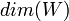$dim(W)$.

First, let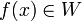$f(x) \in W$. Then we can decompose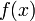$f(x)$ since there is a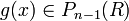$g(x) \in P_{n - 1}(R)$ such that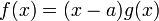$f(x) = (x - a)g(x)$. From here, there are several approaches:

Approach 1: Use Isomorphisms

We show that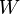$W$ is isomorphic to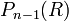$P_{n - 1}(R)$. Let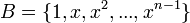$B = \{1, x, x^2, ..., x^{n - 1}\}$ be the standard ordered basis of$P_{n - 1}(R)$ and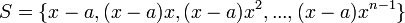$S = \{x - a, (x - a)x, (x - a)x^2, ..., (x - a)x^{n - 1}\}$ be a subset of$W$. Then there is a unique linear transformation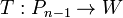$T:P_{n - 1} \to W$ such that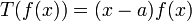$T(f(x)) = (x - a)f(x)$ where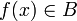$f(x) \in B$. Show that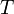$T$ is both one-to-one and onto and conclude that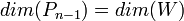$dim(P_{n - 1}) = dim(W)$.

Approach 2: Use the Rank-Nullity Theorem

Let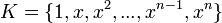$K = \{1, x, x^2, ..., x^{n - 1}, x^n\}$ be the standard ordered basis of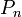$P_n$ and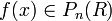$f(x) \in P_{n}(R)$. Then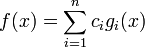$f(x) = \displaystyle\sum_{i=1}^{n} c_ig_i(x)$ where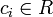$c_i \in R$ and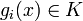$g_i(x) \in K$. Define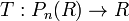$T: P_{n}(R) \to R$ by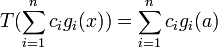$T(\displaystyle\sum_{i=1}^{n} c_ig_i(x))= \displaystyle\sum_{i=1}^{n} c_ig_i(a)$. Then it is easy to show that$T$ is both well-defined and linear. Afterwards, show that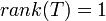$rank(T) = 1$ and use the rank-nullity theorem to conclude that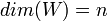$dim(W) = n$.

Approach 3: Find a Basis with the Decomposed Polynomial

This approach is straightforward. Show that$S = \{x - a, (x - a)x, (x - a)x^2, ..., (x - a)x^{n - 1}\}$ is a basis of$W$.

Approach 4: Find a Basis without the Decomposed Polynomial

This approach requires a little more cleverness when constructing the basis: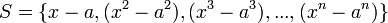$S = \{x - a, (x^2 - a^2), (x^3 - a^3), ..., (x^n - a^n)\}$.

#### Cite Carefully

Boris's Section Only

If you use in your proof Corollary 1 of the Fundamental Theorem of Algebra, then please cite it as "Corollary 1 of the Fundamental Theorem of Algebra". Do not cite it as the "Fundamental Theorem of Algebra" since that means you are citing the fundamental theorem instead of its corollary.